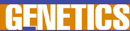# Population Genetics - Research Article from World of Genetics

This encyclopedia article consists of approximately 3 pages of information about Population Genetics.
 This section contains 794 words (approx. 3 pages at 300 words per page) View a FREE samplePopulation genetics is the study of gene variations (alleles) in a population. Allele frequencies within populations can reveal the selective factors and breeding patterns of that population. To determine the true allele frequency of a population, all the individuals in that population must be tested, but this is not practical for most studies. Instead, allele frequencies are usually estimated by testing a sample of individuals from the population, which is representative of the population. How representative this sample is can be tested by mathematics and this sampling math is an important part of population genetics. A powerful tool within this area of research is that of mathematical modeling using computers. Mathematical models are used to predict what effects such things as selection, population size, mutation, and migration have upon the occurrence and frequency of both linked and unlinked genes.

For example, by measuring the allele frequencies...

 This section contains 794 words (approx. 3 pages at 300 words per page) View a FREE sample